`mailto:` blog -at- heyrick -dot- eu

Before we begin... today is wall to wall code with an evolution of a parser to handle evaluating expressions (that means writing a program to read "1 + 1" and perform the calculation).
If that sort of thing isn't your cup of tea, then don't bother reading any more of this blog entry. There's nothing but lots of code here today.

## Expression evaluation with ChatGPT

Something I've wanted to know how to do for a while is to write an expression evaluator. That is to say, if I pass into a program "`2 * (3 + 4) - 5 / 5`" then it should reply with a value according to the rules of the mathematics in use.

• `1.8` (or '1' as an integer):
Left to right only solving brackets first, like:
`3 + 4 = 7; 2 * 7 = 14; 14 - 5 = 9; 9 / 5 = 1.8`
• `0.8` (or '0' as an integer):
`2 * (((3 + 4) - 5) / 5)`
• `12`:
`2 * ((3 + 4) - (5 / 5))`
• `13`:
`(2 * (3 + 4)) - (5 / 5)`

The last value, 13, is the one typically considered correct (it's what BASIC does, for instance) as...

The first thing to solve is the calculation in brackets. `3 + 4 = 7`
Next, we would solve the multiplication. `2 * 7 = 14`.
Next, we skip over the subraction and solve the division, because division has a higher precedence than subtraction. So does multiply, but since it also came first we could just do it.
So, `5 / 5 = 1`.
Finally, we do the subtraction. `14 - 1 = 13`
Nothing left to do, the answer is 13.

Aside: Precedence?
Imagine you have the following calculation: `17 - 5 × 3 + 8 ÷ 2`
Is the answer -2, 6, 22, or something else?
By having some operations (add, subtract, multiply, divide) being more important than others, they get solved first. This is why you can look at that equation and know that the correct answer is 6.
In mathematics, a common mnemonic is BIDMAS which stands for Brackets, Indices, Division, Multiplication, Addition, and Subtraction. However the latter two pairs (×, ÷ and +, -) are evaluated with equal 'priority' reading left to right.

As you can imagine, writing something to cope with this is a little harder than simply looking at the string from left to right and obeying what's there. You can see from the above that we'd be looking at remembering various values and actions to perform on them.

Well, since everybody else is doing it, I asked ChatGPT.

### A simple expression evaluator

Here's what ChatGPT offered:

Sure, I can provide you with a simplified example of C code to handle evaluating expressions with support for mathematical operators, brackets, and operator precedence. This code doesn't cover all edge cases and optimizations but should give you a basic idea of how to approach expression evaluation.

```#include <stdio.h>
#include <stdlib.h>
#include <ctype.h>

// Function to check if a character is an operator
int isOperator(char c) {
return (c == '+' || c == '-' || c == '*' || c == '/');
}

// Function to get the precedence of an operator
int getPrecedence(char op) {
if (op == '+' || op == '-') return 1;
if (op == '*' || op == '/') return 2;
return 0; // Default precedence for non-operators
}

// Function to perform binary operations
int applyOperator(int a, char op, int b) {
switch (op) {
case '+': return a + b;
case '-': return a - b;
case '*': return a * b;
case '/': return a / b;
default:
printf("Invalid operator: %c\n", op);
exit(1);
}
}

// Function to evaluate an expression
int evaluateExpression(const char* expr) {
int stackValues; // Stack to hold values
char stackOperators; // Stack to hold operators
int valIndex = 0;
int opIndex = 0;

for (int i = 0; expr[i]; i++) {
if (expr[i] == ' ') continue; // Skip spaces

if (isdigit(expr[i])) {
// If the character is a digit, parse the number
int num = 0;
while (isdigit(expr[i])) {
num = num * 10 + (expr[i] - '0');
i++;
}
i--; // Decrement i to account for the last digit
stackValues[valIndex++] = num;
} else if (expr[i] == '(') {
// If it's an open parenthesis, push it onto the operator stack
stackOperators[opIndex++] = '(';
} else if (expr[i] == ')') {
// If it's a close parenthesis, pop operators and evaluate until an open parenthesis is encountered
while (opIndex > 0 && stackOperators[opIndex - 1] != '(') {
char op = stackOperators[--opIndex];
int b = stackValues[--valIndex];
int a = stackValues[--valIndex];
stackValues[valIndex++] = applyOperator(a, op, b);
}
// Pop the open parenthesis
opIndex--;
} else if (isOperator(expr[i])) {
// If it's an operator, pop and evaluate operators with higher precedence
while (opIndex > 0 && getPrecedence(stackOperators[opIndex - 1]) >= getPrecedence(expr[i])) {
char op = stackOperators[--opIndex];
int b = stackValues[--valIndex];
int a = stackValues[--valIndex];
stackValues[valIndex++] = applyOperator(a, op, b);
}
// Push the current operator onto the stack
stackOperators[opIndex++] = expr[i];
} else {
printf("Invalid character: %c\n", expr[i]);
exit(1);
}
}

// Evaluate any remaining operators
while (opIndex > 0) {
char op = stackOperators[--opIndex];
int b = stackValues[--valIndex];
int a = stackValues[--valIndex];
stackValues[valIndex++] = applyOperator(a, op, b);
}

// The final result will be at the top of the values stack
return stackValues;
}

int main() {
const char* expression = "2 * (3 + 4) - 5 / 5";
int result = evaluateExpression(expression);
printf("Result: %d\n", result);
return 0;
}```

This code provides a basic framework for evaluating expressions with support for mathematical operators, brackets, and operator precedence. You can use and expand upon this as a starting point.

Compiling it results in this:

```Norcroft RISC OS Arm C vsn 5.89 [18 Feb 2022]
"GPTStuff:c.one", line 28: Warning: implicit return in non-void function
"GPTStuff:c.one", line 90: Warning: Old-style function 'main'
GPTStuff:c.one: 2 warnings, 0 errors, 0 serious errors```

And running it?

`Result: 13`

Result!

It was also interesting to see how it was actually working its magic. Let's break it down.

Given `2 * (3 + 4) - 5 / 5`, we would solve it like this:

• `2` - remember this (number stack)
• `*` - remember this (operator stack)
• `(` - remember this (operator stack)
• `3` - remember this (number stack)
• `+` - remember this (operator stack)
• `4` - remember this (number stack)
• `)` - okay, pull one operation (the '`+`') and two numbers (3 and 4).
Perform the calculation. Remember the result (put 7 on the number stack).
• Is the operation on the stack a '('?
It is, so pull it off the operator stack and discard it, brackets dealt with.
• `-` - as we have two operators now (the `-` and the `*` on the stack), take a look to see if the other is more important.
It is, so pull one operation ('`*`') and two numbers (2 and 7). Perform the calculation. Remember the result (put 14 on the number stack).
And remember the '`-`' (operator stack).
• `5` - remember this (number stack).
• `/` - as we have two operators now (the `/` and the `-` on the stack), take a look to see if the other is more important.
It is not, so remember the '`/`' (operator stack).
• `5` - remember this (number stack)
• END OF INPUT.
• There's still stuff on the operator stack, so pull one operation (the '`/`') and two numbers (5 and 5).
Perform the calculation. Remember the result (put 1 on the number stack).
• There's still stuff on the operator stack, so pull one operation (the '`-`') and two numbers (14 and 1).
Perform the calculation. Remember the result (put 13 on the number stack)..
• There is nothing left on the operator stack, so we're finished and the value in the number stack is the answer.
• The answer is, therefore, 13.

This alone was enough to show me where I was going wrong in my thoughts of how to approach this sort of thing. I was dramatically overthinking it. Oops.

But... it's a little simplistic, isn't it? Since we have a friendly and compliant bot to do the hard work, let's ask for a little more.

### Adding binary shifting and logical operators

I asked how it would implement shifting and logical operators, that is to say "1 << 2" to shift 1 two places to the left (which makes it become 4) as well as logical operations such as AND and OR; such as 123 AND 15 (which equals 11).
If you aren't sure how I got those results, head over to your favourite search engine to brush up on binary mathematics.

ChatGPT said:

Adding support for binary shifting and logical operators like AND in your expression evaluator involves extending the isOperator(), getPrecedence(), and applyOperator() functions to handle these operators. Here's an updated version of the code to include support for these operators:

```#include <stdio.h>
#include <stdlib.h>
#include <ctype.h>

// Function to check if a character is an operator
int isOperator(char c) {
return (c == '+' || c == '-' || c == '*' || c == '/' || c == '&' || c == '|' || c == '^' || c == '<<' || c == '>>');
}

// Function to get the precedence of an operator
int getPrecedence(char op) {
switch (op) {
case '+':
case '-':
return 1;
case '*':
case '/':
return 2;
case '&':
return 3;
case '|':
return 4;
case '^':
return 5;
case '<<':
case '>>':
return 6;
default:
return 0; // Default precedence for non-operators
}
}

// Function to perform binary operations
int applyOperator(int a, char op, int b) {
switch (op) {
case '+': return a + b;
case '-': return a - b;
case '*': return a * b;
case '/': return a / b;
case '&': return a & b;
case '|': return a | b;
case '^': return a ^ b;
case '<<': return a << b;
case '>>': return a >> b;
default:
printf("Invalid operator: %c\n", op);
exit(1);
}
}

// Rest of the code remains the same
// ...```

With these modifications, your expression evaluator can now handle binary shifting and logical operators like AND, OR, and XOR along with the mathematical operators. You can use these operators in your expressions as needed.

Okay, I'll need to paste bits together. No big, I've got this.

But it won't work. Why? Well, how does one match "<<" as a single character?

As expected:

```Norcroft RISC OS Arm C vsn 5.89 [18 Feb 2022]
"GPTStuff:c.two", line 7: Warning: non-portable - not 1 char in '...'
"GPTStuff:c.two", line 7: Warning: non-portable - not 1 char in '...'
"GPTStuff:c.two", line 25: Warning: non-portable - not 1 char in '...'
"GPTStuff:c.two", line 26: Warning: non-portable - not 1 char in '...'
"GPTStuff:c.two", line 43: Warning: non-portable - not 1 char in '...'
"GPTStuff:c.two", line 44: Warning: non-portable - not 1 char in '...'
"GPTStuff:c.two", line 49: Warning: implicit return in non-void function
"GPTStuff:c.two", line 111: Warning: Old-style function 'main'
GPTStuff:c.two: 8 warnings, 0 errors, 0 serious errors```

It does still give the result as 13, because we're still using the old calculation. If we change it to be `(2 * (3 + 4) - 5 / 5) << 1` then instead of the expected answer of 26, it says:

`Invalid character: <`

### Let's try that again

I called the AI bot on this and...

I apologize for the oversight. You are correct; the right shift operator << consists of two characters. Here's the corrected code snippet to handle binary shifting with two-character operators:

```// Function to check if a character is an operator
int isOperator(const char* op) {
return (strcmp(op, "+") == 0 || strcmp(op, "-") == 0 || strcmp(op, "*") == 0 ||
strcmp(op, "/") == 0 || strcmp(op, "&") == 0 || strcmp(op, "|") == 0 ||
strcmp(op, "^") == 0 || strcmp(op, "<<") == 0 || strcmp(op, ">>") == 0);
}

// Function to get the precedence of an operator
int getPrecedence(const char* op) {
if (strcmp(op, "+") == 0 || strcmp(op, "-") == 0)
return 1;
else if (strcmp(op, "*") == 0 || strcmp(op, "/") == 0)
return 2;
else if (strcmp(op, "&") == 0)
return 3;
else if (strcmp(op, "|") == 0)
return 4;
else if (strcmp(op, "^") == 0)
return 5;
else if (strcmp(op, "<<") == 0 || strcmp(op, ">>") == 0)
return 6;
else
return 0; // Default precedence for non-operators
}

// Rest of the code remains the same
// ...```

Now, the code properly handles two-character binary shift operators like << and >>. You can use these operators in your expressions as needed.

No, that won't work either. Because applyOperator() is still expecting a single character as input, and the operator stack still expects single characters to be stored and retrieved.

Compiling it results in:

```Norcroft RISC OS Arm C vsn 5.89 [18 Feb 2022]
"GPTStuff:c.three", line 41: Warning: non-portable - not 1 char in '...'
"GPTStuff:c.three", line 42: Warning: non-portable - not 1 char in '...'
"GPTStuff:c.three", line 47: Warning: implicit return in non-void function
"GPTStuff:c.three", line 81: Error: <argument 1 to 'isOperator'>: implicit cast of non-0 int to pointer
"GPTStuff:c.three", line 83: Error: <argument 1 to 'getPrecedence'>: implicit cast of non-0 int to pointer
"GPTStuff:c.three", line 83: Error: <argument 1 to 'getPrecedence'>: implicit cast of non-0 int to pointer
"GPTStuff:c.three", line 109: Warning: Old-style function 'main'
GPTStuff:c.three: 4 warnings, 3 errors, 0 serious errors```

So I cast the characters as `char *`, and since I can't be arsed to deal with the two-character issue, I simply make them single characters.

But...

`Invalid character: *`

When I dropped in some trace code, it was getting gibberish passed to isOperator(), so I simply reverted those to accept single characters and did a quick cast to string for the tests. Okay, it wouldn't work with "<<" but since the rest of the code was a bit of a mess with respect to this, I just did enough to get it to work. And it did.

`Result: 26`

Actually, what was originally being passed to isOperator() was "`CE!!!!`". The eagle eyed might notice it as part of the embedded string that now lives at address &0, namely "`ýýýýýýýýýýýýýýýý!!!!NULL POINTER DEREFERENCE!!!!`", so something was going wrong and trying to read from address +30 from the bottom of memory. Quite possibly passing characters cast as strings might be... a tad upsetting to the thing.

I asked how the code would be modified to cater for the use of variables in addition to just numbers.

To modify the code to handle variables with names instead of just numbers, you'll need to introduce a mechanism for variable lookup and replace the variable names in the expression with their corresponding integer values. Here's a modified version of the code that allows for variables:

```#include <stdio.h>
#include <stdlib.h>
#include <ctype.h>
#include <string.h>

// Function to check if a character is an operator
int isOperator(char c) {
return (c == '+' || c == '-' || c == '*' || c == '/' || c == '&' || c == '|' || c == '^' || c == '<<' || c == '>>');
}

// Function to get the precedence of an operator
int getPrecedence(char op) {
switch (op) {
case '+':
case '-':
return 1;
case '*':
case '/':
return 2;
case '&':
return 3;
case '|':
return 4;
case '^':
return 5;
case '<<':
case '>>':
return 6;
default:
return 0; // Default precedence for non-operators
}
}

// Function to perform binary operations
int applyOperator(int a, char op, int b) {
switch (op) {
case '+': return a + b;
case '-': return a - b;
case '*': return a * b;
case '/': return a / b;
case '&': return a & b;
case '|': return a | b;
case '^': return a ^ b;
case '<<': return a << b;
case '>>': return a >> b;
default:
printf("Invalid operator: %c\n", op);
exit(1);
}
}

// Function to evaluate an expression with variable lookup
int evaluateExpression(const char* expr, int (*getVarValue)(const char* varName)) {
int stackValues; // Stack to hold values
char stackOperators; // Stack to hold operators
int valIndex = 0;
int opIndex = 0;

for (int i = 0; expr[i]; i++) {
if (expr[i] == ' ') continue; // Skip spaces

if (isdigit(expr[i])) {
// If the character is a digit, parse the number
int num = 0;
while (isdigit(expr[i])) {
num = num * 10 + (expr[i] - '0');
i++;
}
i--; // Decrement i to account for the last digit
stackValues[valIndex++] = num;
} else if (isalpha(expr[i])) {
// If it's an alphabet character, parse the variable
char varName;
int varNameIndex = 0;
while (isalnum(expr[i])) {
varName[varNameIndex++] = expr[i];
i++;
}
varName[varNameIndex] = '\0';
i--; // Decrement i to account for the last character
int varValue = getVarValue(varName);
if (varValue == -1) {
exit(1);
}
stackValues[valIndex++] = varValue;
} else if (expr[i] == '(') {
// If it's an open parenthesis, push it onto the operator stack
stackOperators[opIndex++] = '(';
} else if (expr[i] == ')') {
// If it's a close parenthesis, pop operators and evaluate until an open parenthesis is encountered
while (opIndex > 0 && stackOperators[opIndex - 1] != '(') {
char op = stackOperators[--opIndex];
int b = stackValues[--valIndex];
int a = stackValues[--valIndex];
stackValues[valIndex++] = applyOperator(a, op, b);
}
// Pop the open parenthesis
opIndex--;
} else if (isOperator(expr[i])) {
// If it's an operator, pop and evaluate operators with higher precedence
while (opIndex > 0 && getPrecedence(stackOperators[opIndex - 1]) >= getPrecedence(expr[i])) {
char op = stackOperators[--opIndex];
int b = stackValues[--valIndex];
int a = stackValues[--valIndex];
stackValues[valIndex++] = applyOperator(a, op, b);
}
// Push the current operator onto the stack
stackOperators[opIndex++] = expr[i];
} else {
printf("Invalid character: %c\n", expr[i]);
exit(1);
}
}

// Evaluate any remaining operators
while (opIndex > 0) {
char op = stackOperators[--opIndex];
int b = stackValues[--valIndex];
int a = stackValues[--valIndex];
stackValues[valIndex++] = applyOperator(a, op, b);
}

// The final result will be at the top of the values stack
return stackValues;
}

// Example function to get variable values (replace with your own implementation)
int getVariableValue(const char* varName) {
if (strcmp(varName, "x") == 0) return 10;
if (strcmp(varName, "y") == 0) return 20;
}

int main() {
const char* expression = "x * (y + 5) - 2";
int result = evaluateExpression(expression, getVariableValue);
printf("Result: %d\n", result);
return 0;
}```

In this code, the evaluateExpression function now supports variables, and you need to provide the getVarValue function, which takes a variable name as input and returns its corresponding integer value. Replace the getVariableValue function with your own implementation that retrieves variable values based on the variable name.

Note, for what it is worth, that it has reverted back to thinking "<<" is a single character.

If x is ten and y is twenty, then the calculation `x * (y + 5) - 2` ought to be 248.

`Result: 248`

I asked ChatGPT to modify the code in order to support evaluations like `this > that`, because while it might be useful to have the expression evaluator support setting values or variables, like:
`  LET myval = x * (y + 5) - 2`
it seems to me that pretty much the same code would be required to handle:
`  IF x > y THEN`
as you'd just evaluate the expression to see if it's zero or non-zero.

To modify the code to support logical evaluation such as comparisons like "x > y" and returning True (1) or False (0) depending on the result, you can add support for comparison operators and modify the evaluateExpression function accordingly. Here's an updated version of the code:

```#include <stdio.h>
#include <stdlib.h>
#include <ctype.h>
#include <string.h>

// Function to check if a character is an operator
int isOperator(const char* op) {
return (strcmp(op, "+") == 0 || strcmp(op, "-") == 0 || strcmp(op, "*") == 0 ||
strcmp(op, "/") == 0 || strcmp(op, "&") == 0 || strcmp(op, "|") == 0 ||
strcmp(op, "^") == 0 || strcmp(op, "<<") == 0 || strcmp(op, ">>") == 0 ||
strcmp(op, "==") == 0 || strcmp(op, "!=") == 0 || strcmp(op, ">") == 0 ||
strcmp(op, "<") == 0 || strcmp(op, ">=") == 0 || strcmp(op, "<=") == 0);
}

// Function to get the precedence of an operator
int getPrecedence(const char* op) {
if (strcmp(op, "+") == 0 || strcmp(op, "-") == 0)
return 1;
else if (strcmp(op, "*") == 0 || strcmp(op, "/") == 0)
return 2;
else if (strcmp(op, "&") == 0)
return 3;
else if (strcmp(op, "|") == 0)
return 4;
else if (strcmp(op, "^") == 0)
return 5;
else if (strcmp(op, "<<") == 0 || strcmp(op, ">>") == 0)
return 6;
else if (strcmp(op, "==") == 0 || strcmp(op, "!=") == 0 ||
strcmp(op, ">") == 0 || strcmp(op, "<") == 0 ||
strcmp(op, ">=") == 0 || strcmp(op, "<=") == 0)
return 7; // Precedence for comparison operators
else
return 0; // Default precedence for non-operators
}

// Function to perform binary operations
int applyOperator(int a, const char* op, int b) {
if (strcmp(op, "+") == 0) return a + b;
else if (strcmp(op, "-") == 0) return a - b;
else if (strcmp(op, "*") == 0) return a * b;
else if (strcmp(op, "/") == 0) return a / b;
else if (strcmp(op, "&") == 0) return a & b;
else if (strcmp(op, "|") == 0) return a | b;
else if (strcmp(op, "^") == 0) return a ^ b;
else if (strcmp(op, "<<") == 0) return a << b;
else if (strcmp(op, ">>") == 0) return a >> b;
else if (strcmp(op, "==") == 0) return a == b;
else if (strcmp(op, "!=") == 0) return a != b;
else if (strcmp(op, ">") == 0) return a > b;
else if (strcmp(op, "<") == 0) return a < b;
else if (strcmp(op, ">=") == 0) return a >= b;
else if (strcmp(op, "<=") == 0) return a <= b;
else {
printf("Invalid operator: %s\n", op);
exit(1);
}
}

// Rest of the code remains the same
// ...

int main() {
const char* expression = "x > y";
int result = evaluateExpression(expression, getVariableValue);
printf("Result: %s\n", result ? "True" : "False");
return 0;
}```

With these modifications, the evaluateExpression function can now handle comparison operators like ==, !=, >, <, >l=, and <=, and it returns True (1) or False (0) based on the result of the comparison. Make sure to replace the getVariableValue function with your own implementation to retrieve variable values based on variable names.

Of course, my compiler took one look at that mess and exploded.

```Norcroft RISC OS Arm C vsn 5.89 [18 Feb 2022]
"GPTStuff:c.five", line 58: Warning: implicit return in non-void function
"GPTStuff:c.five", line 105: Error: <argument 2 to 'applyOperator'>: implicit cast of non-0 int to pointer
"GPTStuff:c.five", line 109: Error: <argument 1 to 'isOperator'>: implicit cast of non-0 int to pointer
"GPTStuff:c.five", line 111: Error: <argument 1 to 'getPrecedence'>: implicit cast of non-0 int to pointer
"GPTStuff:c.five", line 111: Error: <argument 1 to 'getPrecedence'>: implicit cast of non-0 int to pointer
"GPTStuff:c.five", line 115: Error: <argument 2 to 'applyOperator'>: implicit cast of non-0 int to pointer
"GPTStuff:c.five", line 130: Error: <argument 2 to 'applyOperator'>: implicit cast of non-0 int to pointer
"GPTStuff:c.five", line 144: Warning: Old-style function 'main'
GPTStuff:c.five: 2 warnings, 6 errors, 0 serious errors```

I didn't bother to fix this, but instead asked if it could directly handle something like `4 * (x >= y)` (which would return either 0 if x was less than y, or 4).

## A minor rethink

I apologize for the oversight in the previous code. The provided code doesn't support the evaluation of logical expressions directly. To correctly evaluate "4 * (x >= y)" and return either zero or four, you would need to extend the code to support logical expressions explicitly. Here's a modified version of the code to achieve this:

```#include <stdio.h>
#include <stdlib.h>
#include <ctype.h>
#include <string.h>

// Function to check if a character is an operator
int isOperator(const char* op) {
return (strcmp(op, "+") == 0 || strcmp(op, "-") == 0 || strcmp(op, "*") == 0 ||
strcmp(op, "/") == 0 || strcmp(op, "&") == 0 || strcmp(op, "|") == 0 ||
strcmp(op, "^") == 0 || strcmp(op, "<<") == 0 || strcmp(op, ">>") == 0 ||
strcmp(op, "==") == 0 || strcmp(op, "!=") == 0 || strcmp(op, ">") == 0 ||
strcmp(op, "<") == 0 || strcmp(op, ">=") == 0 || strcmp(op, "<=") == 0);
}

// Function to get the precedence of an operator
int getPrecedence(const char* op) {
if (strcmp(op, "+") == 0 || strcmp(op, "-") == 0)
return 1;
else if (strcmp(op, "*") == 0 || strcmp(op, "/") == 0)
return 2;
else if (strcmp(op, "&") == 0)
return 3;
else if (strcmp(op, "|") == 0)
return 4;
else if (strcmp(op, "^") == 0)
return 5;
else if (strcmp(op, "<<") == 0 || strcmp(op, ">>") == 0)
return 6;
else if (strcmp(op, "==") == 0 || strcmp(op, "!=") == 0 ||
strcmp(op, ">") == 0 || strcmp(op, "<") == 0 ||
strcmp(op, ">=") == 0 || strcmp(op, "<=") == 0)
return 7; // Precedence for comparison operators
else
return 0; // Default precedence for non-operators
}

// Function to perform binary operations
int applyOperator(int a, const char* op, int b) {
if (strcmp(op, "+") == 0) return a + b;
else if (strcmp(op, "-") == 0) return a - b;
else if (strcmp(op, "*") == 0) return a * b;
else if (strcmp(op, "/") == 0) return a / b;
else if (strcmp(op, "&") == 0) return a & b;
else if (strcmp(op, "|") == 0) return a | b;
else if (strcmp(op, "^") == 0) return a ^ b;
else if (strcmp(op, "<<") == 0) return a << b;
else if (strcmp(op, ">>") == 0) return a >> b;
else if (strcmp(op, "==") == 0) return a == b;
else if (strcmp(op, "!=") == 0) return a != b;
else if (strcmp(op, ">") == 0) return a > b;
else if (strcmp(op, "<") == 0) return a < b;
else if (strcmp(op, ">=") == 0) return a >= b;
else if (strcmp(op, "<=") == 0) return a <= b;
else {
printf("Invalid operator: %s\n", op);
exit(1);
}
}

// Function to evaluate an expression with variable lookup
int evaluateExpression(const char* expr, int (*getVarValue)(const char* varName)) {
int stackValues; // Stack to hold values
const char* stackOperators; // Stack to hold operators
int valIndex = 0;
int opIndex = 0;

for (int i = 0; expr[i]; i++) {
if (expr[i] == ' ') continue; // Skip spaces

if (isdigit(expr[i])) {
// If the character is a digit, parse the number
int num = 0;
while (isdigit(expr[i])) {
num = num * 10 + (expr[i] - '0');
i++;
}
i--; // Decrement i to account for the last digit
stackValues[valIndex++] = num;
} else if (isalpha(expr[i])) {
// If it's an alphabet character, parse the variable
char varName;
int varNameIndex = 0;
while (isalnum(expr[i])) {
varName[varNameIndex++] = expr[i];
i++;
}
varName[varNameIndex] = '\0';
i--; // Decrement i to account for the last character
int varValue = getVarValue(varName);
if (varValue == -1) {
exit(1);
}
stackValues[valIndex++] = varValue;
} else if (expr[i] == '(') {
// If it's an open parenthesis, push it onto the operator stack
stackOperators[opIndex++] = "(";
} else if (expr[i] == ')') {
// If it's a close parenthesis, pop operators and evaluate until an open parenthesis is encountered
while (opIndex > 0 && strcmp(stackOperators[opIndex - 1], "(") != 0) {
const char* op = stackOperators[--opIndex];
int b = stackValues[--valIndex];
int a = stackValues[--valIndex];
stackValues[valIndex++] = applyOperator(a, op, b);
}
// Pop the open parenthesis
opIndex--;
} else if (isOperator(expr + i)) {
// If it's an operator, pop and evaluate operators with higher precedence
const char* op = expr + i;
while (opIndex > 0 && getPrecedence(stackOperators[opIndex - 1]) >= getPrecedence(op)) {
op = stackOperators[--opIndex];
int b = stackValues[--valIndex];
int a = stackValues[--valIndex];
stackValues[valIndex++] = applyOperator(a, op, b);
}
// Push the current operator onto the stack
stackOperators[opIndex++] = op;
// Skip past the operator in the expression
while (isOperator(expr + i)) {
i++;
}
i--; // Decrement i to account for the last character
} else {
printf("Invalid character: %c\n", expr[i]);
exit(1);
}
}

// Evaluate any remaining operators
while (opIndex > 0) {
const char* op = stackOperators[--opIndex];
int b = stackValues[--valIndex];
int a = stackValues[--valIndex];
stackValues[valIndex++] = applyOperator(a, op, b);
}

// The final result will be at the top of the values stack
return stackValues;
}

// Example function to get variable values (replace with your own implementation)
int getVariableValue(const char* varName) {
if (strcmp(varName, "x") == 0) return 10;
if (strcmp(varName, "y") == 0) return 20;
}

int main() {
const char* expression = "4 * (x >= y)";
int result = evaluateExpression(expression, getVariableValue);
printf("Result: %d\n", result);
return 0;
}```

With these modifications, the code evaluates any remaining operators and returns the final result. The example expression "4 * (x >= y)" will correctly evaluate and return either zero or four based on the comparison "x >= y." Make sure to replace the getVariableValue function with your own implementation to retrieve variable values based on variable names.

Except... it doesn't.

`Invalid character: *`

Dropping in a quick printf() shows the problem.

```isOperator -> "* (x >= y)"
Invalid character: *```

It's trying to perform a string match on the entire rest of the string. This is because the only real difference between this and the previous code was to drop in some stuff like `const char* op = expr + i;` which sets 'op' to point to that place in the string, but you can't then match it with anything as the entire rest of the string follows.

Okay, enough of this. I've got the idea of what it's trying to do. Time to introduce an actual intelligence (even if I've spent a lifetime trying to convince people of the opposite).

### I did it myyyy waaayyyyy!

With ChatGPT providing a basic framework of how an expression evaluator should work, time to take these concepts and create my own working expression code. Unlike ChatGPT, I won't say "it works" until it can be shown to do so.

The first thing I did was to translate the operators into tokens. These tokens can fit into a character sized array.
After a little bit of tidying up of the code, and removing all those superfluous `const`s, we now have a functional expression evaluator.

Here's my code:

```/* Expression parser
=================

This code was written by ChatGPT version 3.5,
fixed, revised and improved by Rick Murray version 1.

(C) 2023 Richard Murray

You may use this code in any non-commercial [*] software product.

https://heyrick.eu/blog/index.php?diary=20230920

* - My definition of "commercial" is "it makes you money".
it's commercial, even if you get paid mere pennies.
*/

#include <stdio.h>
#include <stdlib.h>
#include <ctype.h>
#include <string.h>

#define _UNKNOWN    0
#define _SUBTRACT   2
#define _MULTIPLY   3
#define _DIVIDE     4
#define _AND        5
#define _OR         6
#define _EOR        7
#define _SHIFTLEFT  8
#define _SHIFTRIGHT 9
#define _EQUALS     10
#define _NOTEQUALS  11
#define _GREATER    12
#define _LESSER     13
#define _GREATEREQ  14
#define _LESSEREQ   15

int is_operator(char *expr, int *i, int update)
{
// Check if a character is an operator.
// Returns operator token (or zero); may update 'i' if update set.

int  index = *i;
char op = expr[index];
char nextop = expr[index + 1];

switch ( op )
{
break;

case '-' : reply = _SUBTRACT;
break;

case '*' : reply = _MULTIPLY;
break;

case '/' : reply = _DIVIDE;
break;

case '&' : reply = _AND;
break;

case '|' : reply = _OR;
break;

case '^' : reply = _EOR;
break;

case '<' : reply = _LESSER;
switch ( nextop )
{
case '<' : reply = _SHIFTLEFT;
if ( update )
*i = index + 1;
break;

case '=' : reply = _LESSEREQ;
if ( update )
*i = index + 1;
break;
}
break;

case '>' : reply = _GREATER;
switch ( nextop )
{
case '>' : reply = _SHIFTRIGHT;
if ( update )
*i = index + 1;
break;

case '=' : reply = _GREATEREQ;
if ( update )
*i = index + 1;
break;
}
break;

case '=' : if ( nextop == '=' )
{
if ( update )
*i = index + 1;
}
break;

case '!' : if ( nextop == '=' )
{
if ( update )
*i = index + 1;
}
break;
}

}

int get_precedence(char op)
{
// Return the precedence of an operator
switch ( op )
{
case _ADD        : // falls through
case _SUBTRACT   : return 1;

case _MULTIPLY   : // falls through
case _DIVIDE     : return 2;

case _AND        : return 3;

case _OR         : return 4;

case _EOR        : return 5;

case _SHIFTLEFT  : // falls through
case _SHIFTRIGHT : return 6;

case _EQUALS     : // falls through
case _NOTEQUALS  :
case _GREATER    : // (a lot)
case _LESSER     :
case _GREATEREQ  :
case _LESSEREQ   : return 7;
}

return 0; // default precedence for non-operators
}

int apply_operator(int a, char op, int b)
{
// Perform the operations

switch ( op )
{
case _ADD        : return ( a + b );

case _SUBTRACT   : return ( a - b );

case _MULTIPLY   : return ( a * b );

case _DIVIDE     : return ( a / b );

case _AND        : return ( a & b ); // is NOT logical AND

case _OR         : return ( a | b ); // is NOT logical OR

case _EOR        : return ( a ^ b );

case _SHIFTLEFT  : return ( a << b );

case _SHIFTRIGHT : return ( a >> b );

case _EQUALS     : return ( a == b );

case _NOTEQUALS  : return ( a != b );

case _GREATER    : return ( a > b );

case _LESSER     : return ( a < b );

case _GREATEREQ  : return ( a >= b );

case _LESSEREQ   : return ( a <= b );
}

printf("Invalid operator %d.\n", op);
exit(1);

return 0; // keep the compiler happy...
}

int get_variable_value(char *varName)
{
// Look up/return variables - here 'x' and 'y' are baked in
if (strcmp(varName, "x") == 0) return 10;
if (strcmp(varName, "y") == 0) return 20;

}

int evaluate_expression(char *expr, int trace)
{
// Evaluate the expression

int stackValues; // Stack to hold values
char stackOperators; // Stack to hold operators
int valIndex = 0;
int opIndex = 0;

for (int i = 0; expr[i]; i++)
{
if (expr[i] == ' ')  continue; // Skip spaces
if (expr[i] == '\n') continue; // Because fgets()

if ( trace )
printf("Examining input \'%c\' (index = %d)\n", expr[i], i);

if ( isdigit(expr[i]) )
{
// If the character is a digit, parse the number
int num = 0;
while ( isdigit(expr[i]) )
{
num = num * 10 + (expr[i] - '0');
i++;
}
i--; // Decrement i to account for the last digit
if ( trace )
printf("Pushing number %d to stack in position %d.\n", num, valIndex);
stackValues[valIndex++] = num;
}
else if ( isalpha(expr[i]) )
{
// If it's an alphabet character, parse the variable
char varName;
int varNameIndex = 0;
while ( isalnum(expr[i]) )
{
varName[varNameIndex++] = expr[i];
i++;
}
varName[varNameIndex] = '\0';
i--; // Decrement i to account for the last character

int varValue = get_variable_value(varName);
if (varValue == -1)
{
exit(1);
}
if ( trace )
printf("Pushing value of %s (%d) to stack in position %d.\n", varName, varValue, valIndex);
stackValues[valIndex++] = varValue;

}
else if (expr[i] == '(')
{
// If it's an open parenthesis, push it onto the operator stack
if ( trace )
printf("Pushing '(' to opstack in position %d.\n", opIndex);
stackOperators[opIndex++] = '(';
}
else if (expr[i] == ')')
{
// If it's a close parenthesis, pop operators and evaluate until an open parenthesis is encountered
if ( trace )
printf("Input is ')', deal with bracketed code.\n");
while (opIndex > 0 && (stackOperators[opIndex - 1] != '(') )
{
char op = stackOperators[--opIndex];
int b = stackValues[--valIndex];
int a = stackValues[--valIndex];
if ( trace )
printf("BRACKET: Performing operation %d with values %d and %d (result = ", op, a, b);
stackValues[valIndex++] = apply_operator(a, op, b);
if ( trace )
printf("%d)\n", stackValues[valIndex - 1]);
}
// Pop the open parenthesis
opIndex--;

}
else if ( is_operator(expr, &i, 0) )
{
// If it's an operator, pop and evaluate operators with higher precedence
char op = is_operator(expr, &i, 0); // needs a token
if ( trace )
printf("Input is an operator, checking precedence with previous.\n");
while (opIndex > 0 && get_precedence(stackOperators[opIndex - 1]) >= get_precedence(op))
{
op = stackOperators[--opIndex];
int b = stackValues[--valIndex];
int a = stackValues[--valIndex];
if ( trace )
printf("PRECEDENCE: Performing operation %d with values %d and %d (result = ", op, a, b);
stackValues[valIndex++] = apply_operator(a, op, b);
if ( trace )
printf("%d)\n", stackValues[valIndex - 1]);
}
// Push the current operator onto the stack
stackOperators[opIndex++] = is_operator(expr, &i, 1); // get its token
if ( trace )
printf("Pushed operator token %d to OpStack position %d.\n",
stackOperators[opIndex - 1], opIndex - 1);
}
else
{
printf("Invalid character: %c\n", expr[i]);
exit(1);
}
}

// Evaluate any remaining operators
while (opIndex > 0)
{
char op = stackOperators[--opIndex];
int b = stackValues[--valIndex];
int a = stackValues[--valIndex];
if ( trace )
printf("REMAINING: Performing operation %d with values %d and %d (result = ", op, a, b);
stackValues[valIndex++] = apply_operator(a, op, b);
if ( trace )
printf("%d)\n", stackValues[valIndex - 1]);
}

// The final result will be at the top of the values stack
return stackValues;
}

int main(void)
{
char defaultexp[] = "2 * (3 + 4) - y / x";
char expression = "";
int  trace = 0;
int  result = 0;

printf("Default expression is: %s\n", defaultexp);
printf("Enter expression (up to 23 characters), or Return for default. x = 10, y = 20.\n");
printf(": ");
fgets(expression, 24, stdin);
if ( expression == '\n' )
strcpy(expression, defaultexp);

printf("Would you like tracing? (y/N) ");
trace = fgetc(stdin);
if ( ( trace == 'y' ) || ( trace == 'Y' ) )
trace = 1;
else
trace = 0;

result = evaluate_expression(expression, trace);
printf("Result: %d\n", result);

return 0;
}```

It could do with more tidying up, but as it is it builds with no errors or warnings.

The minimal use is just to press `Enter` twice to use the default expression without any tracing.

```Default expression is: 2 * (3 + 4) - y / x
Enter expression (up to 23 characters), or Return for default. x = 10, y = 20.
:
Would you like tracing? (y/N)
Result: 12```

Feel free to enter your own expressions. Remember, though, it's clipped at 23 characters.

```Default expression is: 2 * (3 + 4) - y / x
Enter expression (up to 23 characters), or Return for default. x = 10, y = 20.
: 2*(5*(5*3)+2)+(5*3*3)
Would you like tracing? (y/N) n
Result: 199```

And, finally, if you want to see how it actually does what it does, turn on the tracing. I've made a little animation of the stack behaviours to go alongside the description.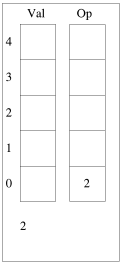```Default expression is: 2 * (3 + 4) - y / x
Enter expression (up to 23 characters), or Return for default. x = 10, y = 20.
:
Would you like tracing? (y/N) y
Examining input '2' (index = 0)
Pushing number 2 to stack in position 0.
Examining input '*' (index = 2)
Input is an operator, checking precedence with previous.
Pushed operator token 3 to OpStack position 0.
Examining input '(' (index = 4)
Pushing '(' to opstack in position 1.
Examining input '3' (index = 5)
Pushing number 3 to stack in position 1.
Examining input '+' (index = 7)
Input is an operator, checking precedence with previous.
Pushed operator token 1 to OpStack position 2.
Examining input '4' (index = 9)
Pushing number 4 to stack in position 2.
Examining input ')' (index = 10)
Input is ')', deal with bracketed code.
BRACKET: Performing operation 1 with values 3 and 4 (result = 7)
Examining input '-' (index = 12)
Input is an operator, checking precedence with previous.
PRECEDENCE: Performing operation 3 with values 2 and 7 (result = 14)
Pushed operator token 2 to OpStack position 0.
Examining input 'y' (index = 14)
Pushing value of y (20) to stack in position 1.
Examining input '/' (index = 16)
Input is an operator, checking precedence with previous.
Pushed operator token 4 to OpStack position 1.
Examining input 'x' (index = 18)
Pushing value of x (10) to stack in position 2.
REMAINING: Performing operation 4 with values 20 and 10 (result = 2)
REMAINING: Performing operation 2 with values 14 and 2 (result = 12)
Result: 12```

### In summary

While I think ChatGPT creates code of dubious quality and does need prompting and/or fixing by a human, I can certainly see it as a useful tool to spark the imagination and help the process of creativity. Last summer, or was it the one before? I tried to write an expression parser. Days later, a great hulking mess that didn't work and is best forgotten.
Today? Thanks to ChatGPT showing me a different way than what I had created (and, actually, rather simpler too!), I had myself some functioning code in an hour or so. Much of the time wasted spent on this today was marking up this blog article as I was going along.

I don't envisage AI replacing programmers just yet, but I can see it as being a potentially useful assistant provided that the programmers in question recognise its limitations and errors and know how to fix them, and don't treat it like StackOverflow to copy-paste stuff that they can't be bothered to do themselves.

Please note that while I check this page every so often, I am not able to control what users write; therefore I disclaim all liability for unpleasant and/or infringing and/or defamatory material. Undesired content will be removed as soon as it is noticed. By leaving a comment, you agree not to post material that is illegal or in bad taste, and you should be aware that the time and your IP address are both recorded, should it be necessary to find out who you are. Oh, and don't bother trying to inline HTML. I'm not that stupid! ☺ ADDING COMMENTS DOES NOT WORK IF READING TRANSLATED VERSIONS.

You can now follow comment additions with the comment RSS feed. This is distinct from the b.log RSS feed, so you can subscribe to one or both as you wish.

 J.G.Harston, 21st September 2023, 00:19 Recursion is your friend. When I wrote the expression evaluator in PDP11 BASIC, it just flowed naturally as parse this bit, if nextchar is lower priority RTS else JSR, loop. See mdfs.net/Software/PDP11/BBCBasic/bbcpdp.zip > usr/src/bbcbasic/Evaluate David Pilling, 21st September 2023, 02:23 "This code was written by ChatGPT" I got told off somewhere else for saying that ChatGPT can only produce code because that code exists somewhere on the web. "Get Real" said the expert "this is generative AI".  In OvationPro you'll find a couple of expression evaluation examples, one in the script language which does all the C stuff of logical operators etc. and another one for evaluating what people type into icons, handles units as well as arithmetic operators. Mine are based on Niklaus Wirth's book "Algorithms+Data structures=Programs" - I think it is a nicer technique than the one above. JGH, 21st September 2023, 09:09 Useful, I'll have to check Wirth and see if I can improve my code. Gavin Wraith, 21st September 2023, 11:16 The FORTH-WRITE take on this is that conventional mathematical notation evolved over many centuries and is quite a mess. Hence the need for a lot of parsing to make up for this. The trouble is that maths has a reputation for icy logic, so the mess comes as a bit of a shock to those who believe that reputation. C Ferris, 21st September 2023, 12:19 Rick using a Bot as a teacher!  It would be useful if the Bot recommended some books:-)

 Add a comment (v0.11) [help?] . . . try the comment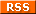feed! Your name Your email (optional) Validation Are you real? Please type 72849 backwards. Your comment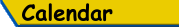Mon Tue Wed Thu Fri Sat Sun «   September 2023   » 1 2 3 4 5 6 7 8 9 10 11 12 13 14 15 16 17 18 19 20 21 22 23 24 25 26 27 28 29 30

(Felicity? Marte? Find out!)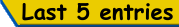List all b.log entries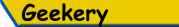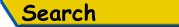Search Rick's b.log!
PS: Don't try to be clever.
It's a simple substring match.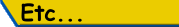Last read at 14:35 on 2023/12/04.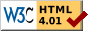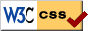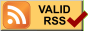© 2023 Rick Murray This web page is licenced for your personal, private, non-commercial use only. No automated processing by advertising systems is permitted. RIPA notice: No consent is given for interception of page transmission.

 Have you noticed the watermarks on pictures? Read the explanation.
Next entry - 2023/09/21